Download post as jupyter notebook

In this tutorial we introduce our library for creating differentiable optimization layers in PyTorch and TensorFlow. You can find more details in:

1. Our NeurIPS 2019 paper
2. Our cvxgrp/cvxpylayers repo and our other PyTorch and TensorFlow examples
3. CVXPY and Disciplined Parametrized Programming

Optimization layers add domain-specific knowledge or learnable hard constraints to machine learning models. Many of these layers solve convex and constrained optimization problems of the form

with parameters $\theta$, objective $f$, and constraint functions $g,h$ and do end-to-end learning through them with respect to $\theta$.

In this tutorial we introduce our new library cvxpylayers for easily creating differentiable new convex optimization layers. This lets you express your layer with the CVXPY domain specific language as usual and then export the CVXPY object to an efficient batched and differentiable layer with a single line of code. This project turns every convex optimization problem expressed in CVXPY into a differentiable layer. Before this, implementing these layers has required manually implementing efficient problem-specific batched solvers and manually implicitly differentiating the optimization problem. Most prior work on differentiable optimization layers has used PyTorch and in our project we significantly bring differentiable convex optimization layers to TensorFlow so that it is just as easy now.

Overview. After going deeper into the background and motivation of optimization layers, we will present an interpretation of the ReLU, sigmoid, and softmax functions as optimization layers and show how they can be implemented with cvxpylayers. We will then go on to show how to add more sophisticated projection and constraints to neural network layers with cvxpylayers.

# Background and Motivation

See Sections 2 and 3 of our paper for a more complete overview of the related work in this space.

## Domain-specific modeling components for machine learning

Modeling in today’s machine learning landscape typically involves connecting together differentiable components and building block in a way that is end-to-end trainable. Historically and conventionally, practitioners can model with well-known linear operations such as fully connected layers and convolutions composed with non-linear activation functions such as the ReLU and sigmoid. Projections onto the simplex can be done with the softmax function. This modeling approach provides an incredibly expressive function class that can universally approximate any function under mild restrictions. However this expressiveness comes at the cost of trading off model interpretability and data efficiency in many cases. In an attempt to improve this, more sophisticated and specialized modeling components are being proposed, analyzed, and studied in the community that can be integrated with the rest of the machine learning pipeline and learned with standard gradient-based methods.

The role of domain-specific knowledge in the machine learning and computer vision fields has been an active discussion topic over the past decade and beyond. Historically, domain knowledge such as fixed hand-crafted feature and edge detectors were rigidly part of the computer vision pipeline and have been overtaken by learnable convolutional models To highlight the power of convolutional architectures, they provide a reasonable prior for vision tasks even without learning. Machine learning models extend far beyond the reach of vision tasks and the community has a growing interest on domain-specific priors rather than just using fully-connected architectures. These priors ideally can be integrated as end-to-end learnable modules into a larger system that are learned as a whole with gradient-based information. In contrast to pure fully-connected architectures, specialized submodules ideally improve the data efficiency of the model, add interpretability, and enable grey-box verification. More examples include differentiable beam search; differentiable dynamic programming; a differentiable protein simulator; a differentiable particle filter; neural ordinary differential equations and applications to a reversible generative models; relational reasoning on sets, graphs, and trees; geometry-based priors; memory; attention mechanisms; capsule networks; and program synthesis.

## Our focus: Optimization as a layer

In this tutorial, we consider optimization as a layer for machine learning that can be used to inject domain-specific knowledge into the inference procedure. We argue that in addition to optimization’s role in machine learning for parameter optimization or architecture search (neither of which we focus on), optimization should have a third role in machine learning as a modeling component. Optimization is useful because many sophisticated operations can be expressed as optimization problems, and even outside of the field of machine learning optimization is widely used for modeling. Traditional machine learning methods may also fail when trying to learn underlying hard constraints or rules behind a problem that as we will show, are natural to express as parameterized optimization layers.

From another perspective, optimization layers can also be used for partial modeling of optimization problems. Traditionally a modeler needs to have a complete analytic view of their system if they want to use optimization to solve their problem, such as in many control, planning, and scheduling tasks. The techniques we propose lets the practitioner leave latent parts in their optimization-based modeling procedure that can then be learned from data.

# Starting Simple: Convex Optimization Viewpoint of Standard Layers

This tutorial is downloadable as a Jupyter notebook and in the following portion we interleave PyTorch code inline to create all of the plots. Our TensorFlow interface has an identical interface and can be used by replacing torch in the import with tensorflow.

import cvxpy as cp
from cvxpylayers.torch import CvxpyLayer


(You can see the full set of imports and helper functions if you download the notebook backing this post.)

We will start off by showing the variational forms of commonly used ReLU, sigmoid, and softmax functions that interpret them as explicit closed-form solutions to constrained and convex optimization (argmin) problems. Optimization layers provide much more functionality than just subsuming standard activation functions as they can also be parameterized and learned. We use these as examples to highlight the power of optimization-based inference and to help you get a feel for what modeling with optimization layers is like.

The Rectified Linear Unit (ReLU) is an activation function defined by $f(x) = \max\{0, x\}$ and can be easily implemented in PyTorch as:

n = 201
x = torch.linspace(-5, 5, steps=n, requires_grad=True)
y = F.relu(x)
plt.plot(x.detach().numpy(), y.detach().numpy())
plt.title('The Standard ReLU')
plt.xlabel('$x$')
plt.ylabel('$f(x)$')The ReLU is sub-differentiable and a sub-derivative of the ReLU is

We can use PyTorch’s automatic differentiation capabilities to easily obtain this derivative:

y.sum().backward()
plt.plot(to_np(x), to_np(x.grad))
plt.title('The Derivative of the Standard ReLU')
plt.xlabel('$x$')
plt.ylabel('$f\'(x)$')From a variational perspective, we can interpret the ReLU as projecting a point $x\in\mathbb{R}^n$ onto the non-negative orthant as

The usual explicit solution can be obtained by taking the first-order optimality conditions as proved in Section 2.4 here.

Using cvxpylayers, we are able to easily implement this optimization problem as a PyTorch layer. This is powerful since it does not require that our optimization problem has an explicit closed-form solution (even though the ReLU does) and this also shows how easy we can now take this optimization problem and tweak it if we wanted to do so, without having to re-derive the appropriate solution and backwards pass. First we implement the problem as usual with CVXPY:

_x = cp.Parameter(n)
_y = cp.Variable(n)
obj = cp.Minimize(cp.sum_squares(_y-_x))
cons = [_y >= 0]
prob = cp.Problem(obj, cons)


And then use one line to create the PyTorch interface:

layer = CvxpyLayer(prob, parameters=[_x], variables=[_y])


And we can plot what this looks like in the same way as before.

x = torch.linspace(-5, 5, steps=n, requires_grad=True)
y, = layer(x)
plt.plot(to_np(x), to_np(y))
plt.title('The Variational ReLU')
plt.xlabel('$x$')
plt.ylabel('$f(x)$')Since this is computing the same function as the ReLU, we would expect that the derivative looks the same. This is indeed true and we have made it so our optimization layer can be differentiated through just like any other PyTorch layer using normal PyTorch differentiation. Obtaining the derivative in the same way as before through the optimization formulation indeed gives the same result:

y.sum().backward()
plt.plot(to_np(x), to_np(x.grad))
plt.title('The Derivative of the Variational ReLU')
plt.xlabel('$x$')
plt.ylabel('$f\'(x)$')For general optimization problems, we may not be able to write out a closed-form solution like for the ReLU here. From a user’s perspective, these details don’t matter, but under the hood we are implicitly differentiating a set of optimality conditions for this optimization problem, which we’ll go deeper into later.

Similarly, the sigmoid or logistic activation function $f(x) = (1+e^{-x})^{-1}$ can be implemented in PyTorch as:

n = 100
x = torch.linspace(-5, 5, steps=n, requires_grad=True)
y = torch.sigmoid(x)
plt.plot(to_np(x), to_np(y))
plt.title('The Standard Sigmoid')
plt.xlabel('$x$')
plt.ylabel('$f(x)$')The derivative of the sigmoid function is $f’(x)=f(x)(1-f(x))$, which we can again compute with PyTorch’s autodiff:

y.sum().backward()
plt.plot(to_np(x), to_np(x.grad))
plt.title('The Derivative of the Standard Sigmoid')
plt.xlabel('$x$')
plt.ylabel('$f(x)$')From an optimization perspective, the sigmoid projects a point $x\in\mathbb{R}^n$ onto the interior of the unit hypercube as

where $H_b(y) = - \left(\sum_i y_i\log y_i + (1-y_i)\log (1-y_i)\right)$ is the binary entropy function. This can be proved by looking at the KKT conditions as done in Section 2.4 here.

We can also implement this in optimization form as:

_x = cp.Parameter(n)
_y = cp.Variable(n)
obj = cp.Minimize(-_x.T*_y - cp.sum(cp.entr(_y) + cp.entr(1.-_y)))
prob = cp.Problem(obj)
layer = CvxpyLayer(prob, parameters=[_x], variables=[_y])
x = torch.linspace(-5, 5, steps=n, requires_grad=True)
y, = layer(x)
plt.plot(x.detach().numpy(), y.detach().numpy())
plt.title('The Variational Sigmoid')
plt.xlabel('$x$')
plt.ylabel('$f(x)$')We can similarly use PyTorch’s autodiff to differentiate through the the optimization formulation of this layer:

y.sum().backward()
plt.plot(x.detach().numpy(), x.grad.numpy())
plt.title('The Derivative of the Variational Sigmoid')
plt.xlabel('$x$')
plt.ylabel('$f(x)$')Lastly the softmax activation function $f(x)_j = e^{x_j} / \sum_i e^{x_i}$ can be implemented in PyTorch as follows. This time we’ll consider a single vector instead of range of values since the softmax is only interesting in higher dimensions.

d = 5
torch.manual_seed(7)
x = torch.randn(d, requires_grad=True)
y = F.softmax(x, dim=0)

print(f'Input: {to_np(x)}\nOutput: {to_np(y)}')

Input: [-0.1467951   0.7861413   0.94682163 -1.114344    1.6907902 ]
Output: [0.07582434 0.1927426  0.22633949 0.02881426 0.47627935]


We can check to make sure the output sums to 1.

y.sum().item()

1.0


We can also compute the derivative of some output element with respect to all of the inputs. Let’s consider the last element since it’s the largest.

y.backward()

print(f'Gradient: {to_np(x.grad)}')

Gradient: [-0.03611356 -0.09179932 -0.10780083 -0.01372364  0.24943732]


We can again interpret the softmax as projecting a point $x\in\mathbb{R}^n$ onto the interior of the $(n-1)$-simplex $\Delta_{n-1}=\{p\in\mathbb{R}^n\; \vert\; 1^\top p = 1 \; \; {\rm and} \;\; p \geq 0 \}$ as

where $H(y) = -\sum_i y_i \log y_i$ is the entropy function. This is also proved in Section 2.4 here by using the KKT conditions. We can implement the variational form of the softmax with:

_x = cp.Parameter(d)
_y = cp.Variable(d)
obj = cp.Minimize(-_x.T*_y - cp.sum(cp.entr(_y)))
cons = [np.ones(d, dtype=np.float32).T*_y == 1.]
prob = cp.Problem(obj, cons)
layer = CvxpyLayer(prob, parameters=[_x], variables=[_y])

torch.manual_seed(7)
x = torch.randn(d, requires_grad=True)
y, = layer(x)

print(f'Input: {to_np(x)}\nOutput: {to_np(y)}')

Input: [-0.1467951   0.7861413   0.94682163 -1.114344    1.6907902 ]
Output: [0.07582434 0.1927426  0.22633947 0.02881426 0.47627935]


The output is the same and sums to 1:

y.sum().item()

1.0


And the gradient is also the same:

y.backward()

print(f'Gradient: {to_np(x.grad)}')

Gradient: [-0.03611359 -0.09179929 -0.10780083 -0.01372367  0.24943733]


# Even more layers

We next give a few quick examples re-implementing other differentiable projections that have been considered in the literature. For a note on efficiency, solving these problems in conic form with CVXPY may not be the most efficient and that in some cases explicit solutions to these problems also exist that follow from some light analysis. cvxpylayers is still a useful prototyping tool and convenient way of checking the derivative computation in these cases.

## The sparsemax

This is useful for creating sparse predictions in multi-label classification settings and in attention-based neural networks for natural language inference. Even though more efficient implementations exist, in optimization form, the sparsemax does a Euclidean projection onto the simplex as

and can be implemented with:

n = 4
x = cp.Parameter(n)
y = cp.Variable(n)
obj = cp.sum_squares(x-y)
cons = [cp.sum(y) == 1, 0. <= y, y <= 1.]
prob = cp.Problem(cp.Minimize(obj), cons)
layer = CvxpyLayer(prob, [x], [y])

torch.manual_seed(0)
x = torch.randn(n)
y, = layer(x)
print(f'Input: {to_np(x)}\nOutput: {to_np(y)}')

Input: [ 1.5409961  -0.2934289  -2.1787894   0.56843126]
Output: [ 9.8628241e-01 -3.9884393e-10 -8.0997226e-10  1.3717593e-02]


## The csoftmax

This is useful for constraining the outputs of the softmax to be capped at an upper-bound of $u$ and captures the usual softmax if $u=1$ and is useful for sequence tagging. It solves the optimization problem

and can be implemented with:

n, k = 4, 2
u = torch.full([n], 1./k)
x = cp.Parameter(n)
y = cp.Variable(n)
obj = -x*y-cp.sum(cp.entr(y))
cons = [cp.sum(y) == 1., y <= u]
prob = cp.Problem(cp.Minimize(obj), cons)
layer = CvxpyLayer(prob, [x], [y])

torch.manual_seed(0)
x = torch.randn(n)
y, = layer(x)
print(f'Input: {to_np(x)}\nOutput: {to_np(y)}')

Input: [ 1.5409961  -0.2934289  -2.1787894   0.56843126]
Output: [0.5000002  0.1420723  0.02156317 0.33636498]


## The csparsemax

This is the sparse version of the csoftmax and has applications in attention-based translation. It solves the optimization problem

and can be implemented with:

n, k = 4, 2
u = torch.full([n], 1./k)

x = cp.Parameter(n)
y = cp.Variable(n)
obj = cp.sum_squares(x-y)
cons = [cp.sum(y) == 1., 0. <= y, y <= u]
prob = cp.Problem(cp.Minimize(obj), cons)
layer = CvxpyLayer(prob, [x], [y])

torch.manual_seed(0)
x = torch.randn(n)
y, = layer(x)
print(f'Input: {to_np(x)}\nOutput: {to_np(y)}')

Input: [ 1.5409961  -0.2934289  -2.1787894   0.56843126]
Output: [ 5.000000e-01  3.033220e-11 -6.598309e-12  5.000000e-01]


## The Limited Multi-Label Projection (LML) Layer

The LML layer is useful to directly optimize the model’s top-k error or recall in multi-label settings instead of a surrogate. It solves the optimization problem

and can be implemented with:

n, k = 4, 2

x = cp.Parameter(n)
y = cp.Variable(n)
obj = -x*y-cp.sum(cp.entr(y))-cp.sum(cp.entr(1.-y))
cons = [cp.sum(y) == k]
prob = cp.Problem(cp.Minimize(obj), cons)
layer = CvxpyLayer(prob, [x], [y])
torch.manual_seed(0)
x = torch.randn(n)
y, = layer(x)
print(f'Input: {to_np(x)}\nOutput: {to_np(y)}')

Input: [ 1.5409961  -0.2934289  -2.1787894   0.56843126]
Output: [0.8254717  0.43031406 0.10285254 0.64136344]


# Learnable Parametrized Optimization Layers

Up until this point, our toy optimization layers have not been had any learnable components and didn’t add any new modeling functionality. In this part, we will show how to use an optimization layer to learn a parametrized objective function and hard constraints from data that are initially unknown to the model. From a parameter learning perspective, this layer can be interpreted as another differentiable black box and the parameters can be treated just as any other layers’ parameters and learned with gradient-based updates.

## The OptNet QP

We’ll start with the OptNet QP, which in the most general form is expressed as

We can re-implement this using CVXPY with a few lines of code:

n, m, p = 10, 5, 5
Q_sqrt = cp.Parameter((n, n))
q = cp.Parameter(n)
A = cp.Parameter((m, n))
b = cp.Parameter(m)
G = cp.Parameter((p, n))
h = cp.Parameter(p)
x = cp.Variable(n)
obj = cp.Minimize(0.5*cp.sum_squares(Q_sqrt*x) + q.T @ x)
cons = [A @ x == b, G @ x <= h]
prob = cp.Problem(obj, cons)
layer = CvxpyLayer(prob, parameters=[Q_sqrt, q, A, b, G, h], variables=[x])


And we can use the layer with the following. As a caveat that is discussed in the OptNet paper, this QP should be formulated so that it is always feasible. (This may not always happen with the following sampling.)

torch.manual_seed(0)
Q_sqrtval = torch.randn(n, n, requires_grad=True)
qval = torch.randn(n, requires_grad=True)
Aval = torch.randn(m, n, requires_grad=True)
bval = torch.randn(m, requires_grad=True)
Gval = torch.randn(p, n, requires_grad=True)
hval = torch.randn(p, requires_grad=True)
y, = layer(Q_sqrtval, qval, Aval, bval, Gval, hval)
print('Output: ', to_np(y))

Output:  [ 0.33921832  0.3794181   0.2201209  -0.01115552 -0.3120785  -0.22344528
0.5120355  -0.30485314 -0.11274885  0.21256374]


The resulting layer object here solves the QP in conic form and almost has the same performance as the specialized differentiable batched QP library qpth released with OptNet: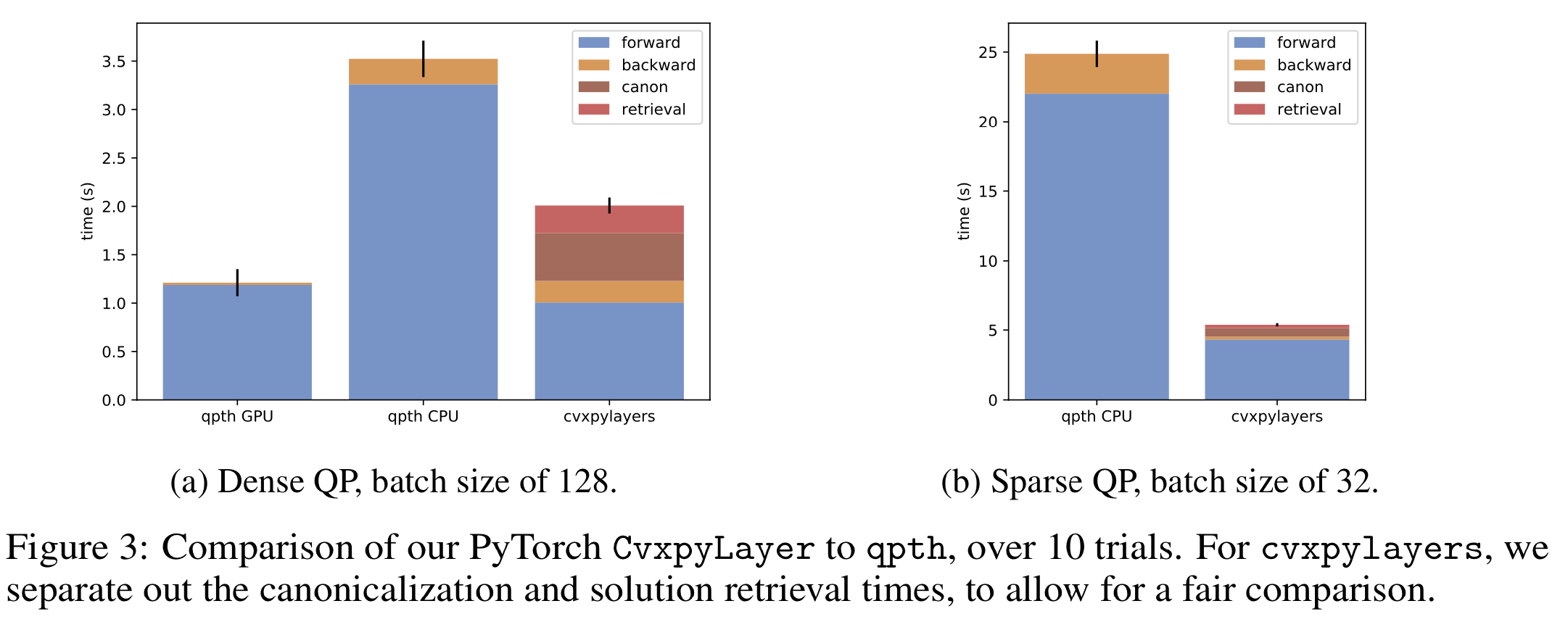Once we add support for differentiating QPs internally to CVXPY the performance will likely be faster than qpth, especially for sparse QPs. Another power of using CVXPY for creating these layers is that you no longer need to manually canonicalize your problems into standard QP form as we show here as qpth required. You can express your problem in a form closer to the math.

## General Polytope Projections

In this next example we’ll look at a simple polytope learning problem of learning a polytope $\{x : Gx \leq h\}$ from data. Suppose we have data generated by projecting points onto some polytope by solving the optimization problem

The solution to this optimization problem does not have an explicit closed-form solution in general and is therefore not as straightforward to integrate as a layer for machine learning. Using CVXPY, we can implement this layer in a few lines of code with:

nx, ncon = 2, 10

_G = cp.Parameter((ncon, nx))
_h = cp.Parameter(ncon)
_x = cp.Parameter(nx)
_y = cp.Variable(nx)
obj = cp.Minimize(0.5*cp.sum_squares(_x-_y))
cons = [_G*_y <= _h]
prob = cp.Problem(obj, cons)

layer = CvxpyLayer(prob, parameters=[_G, _h, _x], variables=[_y])


Note that here, $x$, $G$, and $h$ are all Parameter objects as inputs to the optimization layer, even though we would usually consider $x$ an input to the layer and $G$ and $h$ the parameters.

We can now randomly initialize a polytope as the ground-truth polytope:

torch.manual_seed(6)
G = torch.FloatTensor(ncon, nx).uniform_(-4, 4)
z0 = torch.full([nx], 0.5)
s0 = torch.full([ncon], 0.5)
h = G.mv(z0)+s0
plotConstraints(to_np(G), to_np(h))And we can project a point onto it with:

torch.manual_seed(0)
x = torch.randn(nx)
y, = layer(G, h, x)
print(f'Input: {to_np(x)}\nOutput: {to_np(y)}')

Input: [ 1.5409961 -0.2934289]
Output: [0.6635195  0.34164992]


Next to set up the learning problem we can initialize another polytope that we show here as red:

torch.manual_seed(22)
G_hat = nn.Parameter(torch.FloatTensor(ncon, nx).uniform_(-4, 4).requires_grad_())
h_hat = G_hat.mv(z0)+s0
plotConstraints(to_np(G), to_np(h), to_np(G_hat), to_np(h_hat))Our goal is to now to look at the data to learn the true underlying polytope. In a larger, real-world application, the true, unknown polytope may represent physical or latent constraints on the data that you are trying and you want to try to learn those with an explicit polytope. The following is the usual paradigm of initializing an optimizer to learn this new polytope and then updating it with gradients coming from a loss function. As part of this, we are differentiating the output of the optimization problem with respect to an underlying hard constraint and using this for learning.

opt = torch.optim.Adam([G_hat], lr=1e-2)
losses = []

d = 'polytope_images'
if os.path.exists(d):
shutil.rmtree(d)
os.makedirs(d)

for i in range(2500):
x = torch.randn(nx)
y, = layer(G, h, x)

h_hat = G_hat.mv(z0)+s0
yhat, = layer(G_hat, h_hat, x)
loss = (yhat-y).norm()
losses.append(loss)

if i % 50 == 0:
fig, ax = plotConstraints(to_np(G), to_np(h), to_np(G_hat), to_np(h_hat))
fig.tight_layout()
fig.savefig(f'{d}/{i:04d}.png')
plt.close(fig)

opt.zero_grad()
loss.backward()
opt.step()


We can look at the losses and see that we’ve converged to something reasonable:

fig, ax = plt.subplots(1, 1, figsize=(6,4))
N = 100
xs = np.arange(N-1, len(losses))
ys = np.convolve(losses, np.full(N, 1./N), mode='valid')
ax.plot(xs, ys)
ax.set_xlabel('Iteration')
ax.set_ylabel('Rolling Loss')
ax.set_ylim(0, None)And we can use ImageMagick to create an animation from the frames during training showing our model converging to the true polytope.

os.system(f'convert -delay 10 -loop 0 {d}/????.png {d}/animation.gif');Visualization of the polytope learning during training

Here’s what the learning process looks like for a few other examples: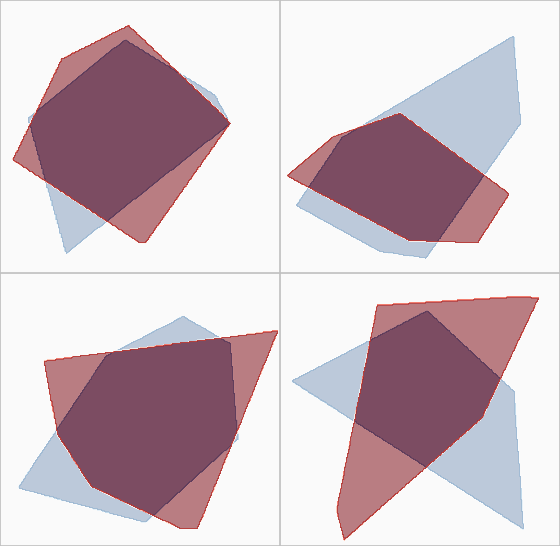More visualizations of the polytope learning during training

A few last comments here: These approximate polytopes are not able to learn how to add new edges since this remains fixed throughout the training process, which you can see happen in the bottom-left. And as a footnote, a convex hull on the projections could be used to solve this problem if you actually care about solving this problem. But this could potentially generate as many edges as there are training data points and our method can be seen as an approximate convex hull fitting approach.

## Ellipsoid Projections

Suppose instead that we want to constrain our latent space to be on an ellipsoid instead of a polytope. Our projection layer now becomes

This is mathematically interesting as it shows an example of differentiating through a non-polyhedral cone program. We can try these layers out by just changing the constraints of our optimization problem:

_A = cp.Parameter((n, n))
_z = cp.Parameter(n)
_x = cp.Parameter(n)
_y = cp.Variable(n)
_t = cp.Variable(n)
obj = cp.Minimize(0.5 * cp.sum_squares(_x - _y))
cons = [0.5 * cp.sum_squares(_A * _t) <= 1, _t == (_y - _z)]
prob = cp.Problem(obj, cons)

layer = CvxpyLayer(prob, parameters=[_A, _z, _x], variables=[_y, _t])


Noting that in this case we needed to manually construct $t=y-z$ for our problem to be DPP-compliant as otherwise we would have been multiplying two Parameter objects. We can similarly visualize the learning process of four ellipsoids: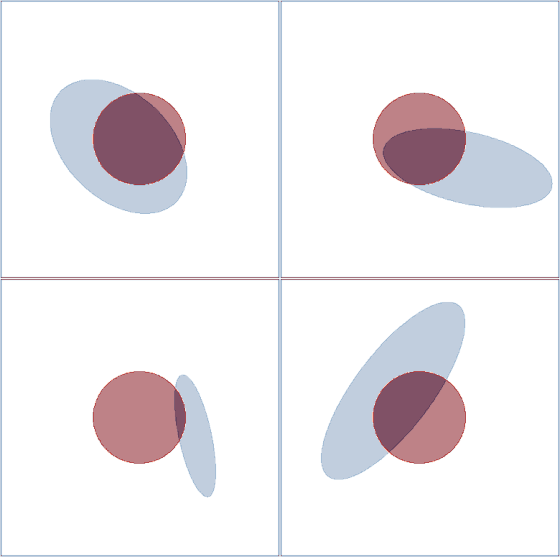Visualization of the ellipsoid learning

# Even more examples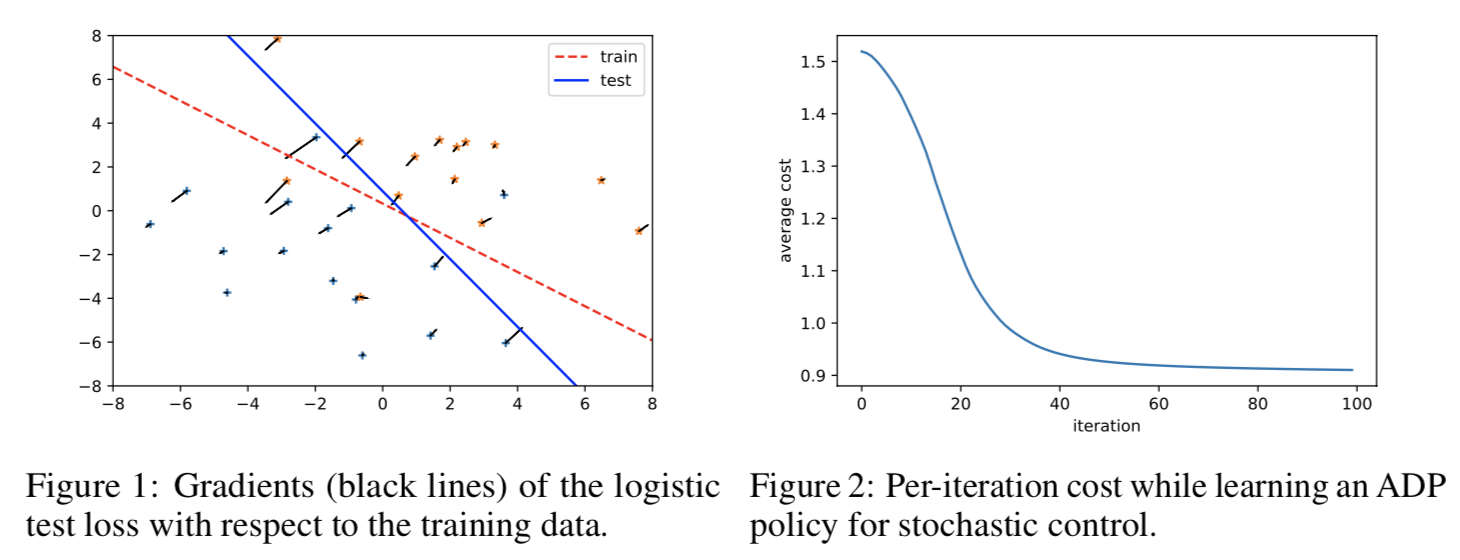In our paper we also show examples in data poisoning and stochastic control. You can find complete notebooks reproducing these examples in our repo and we provide the complete PyTorch implementation and TensorFlow implementation for these experiments, highlighting how easy differentiable convex optimization layers are to use with TensorFlow too.

# How does all of this work?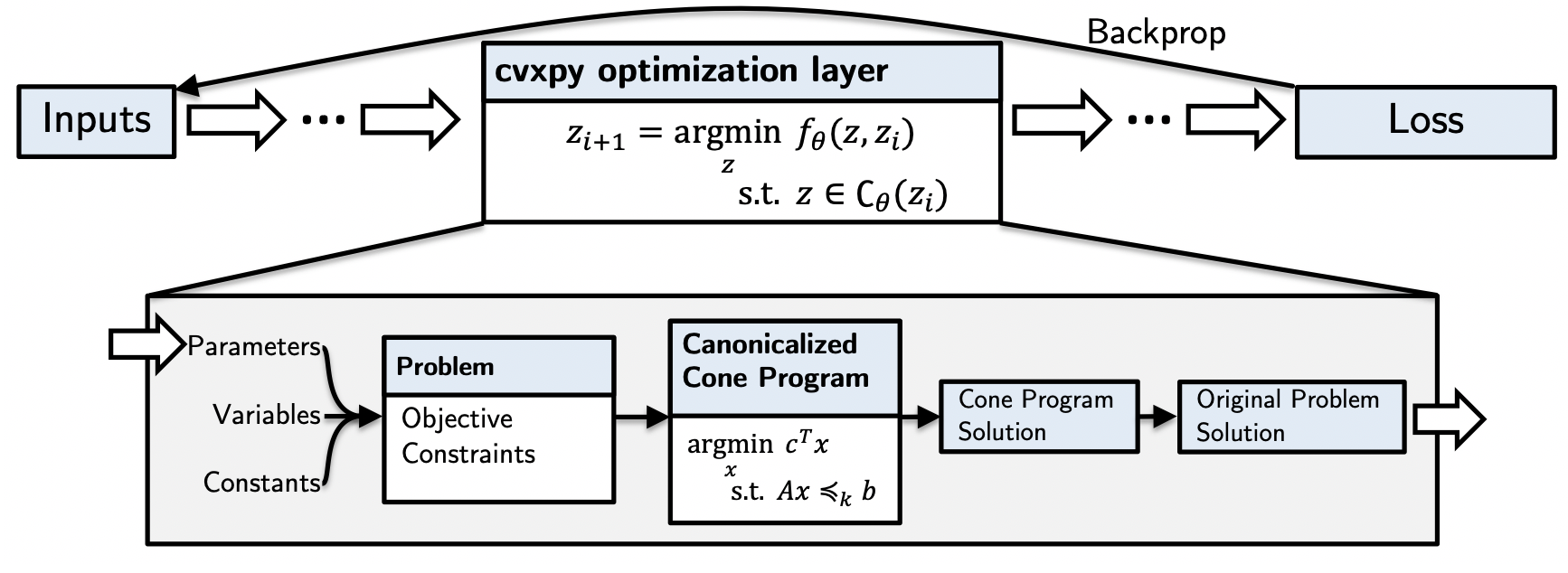You can see the full details in our paper. Our insight is that we can:

1. Map from the CVXPY parameters to an internal cone program (or other canonical representational) in a differentiable way. We have significantly modified CVXPY to do this with an affine map.
2. We can differentiate through the cone program by implicitly differentiating a residual map as discussed in Section 7.3 here and here. This captures KKT differentiation as a special case.
3. We can map from the cone program solution back to the original problem solution in a differentiable way.

We refer to this as affine-solver-affine (ASA) form and can differentiate through every step of it, thus allowing us to create differentiable optimization layers with CVXPY.

# Summary and Future Directions

Our library enables the rapid prototyping of differentiable convex optimization layers in a flexible way that no longer requires manual canonicalization, can be used with PyTorch and TensorFlow, and can efficiently batch up the forward and backward passes. We plan to actively maintain and support this code and are working on adding on even more performance improvements and features. Get in touch if you start using our code and let us know if you have any issues or contributions!

Download post as jupyter notebook